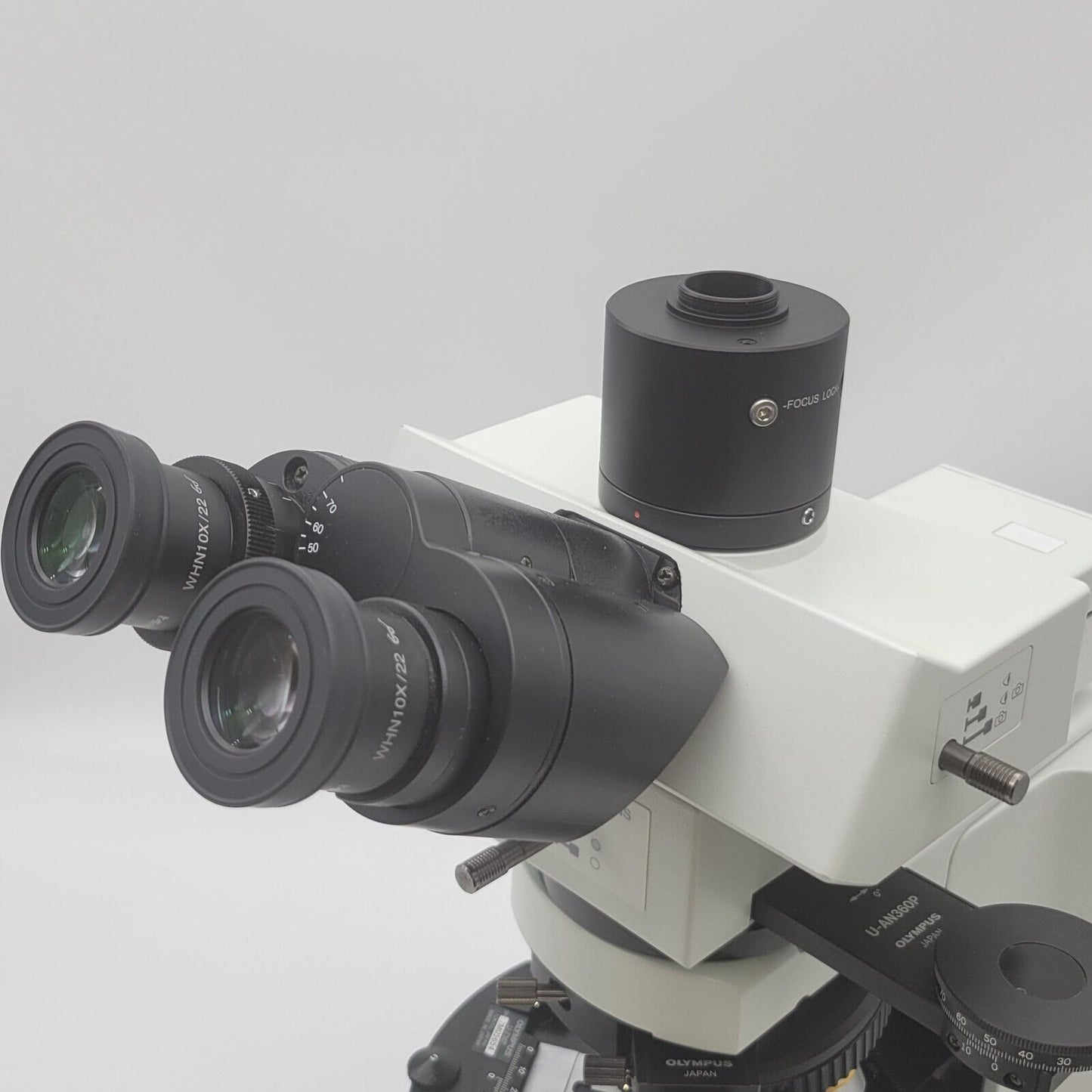# How do I determine the Magnification of my microscope?

The magnification of a microscope is determined by two primary factors: the magnification of the objective lens and the magnification of the eyepiece (ocular). The total magnification is the product of these two magnifications. Here's how you calculate it:

1. Objective Lens Magnification (Nosepiece Magnification): The objective lens is the lens closest to the specimen and provides the initial magnification. The magnification of the objective lens is typically marked on the lens itself (e.g., 4x, 10x, 40x, 100x, etc.). This value indicates how many times larger the image appears compared to the actual size of the specimen.

2. Eyepiece (Ocular) Magnification: The eyepiece is the lens you look through, and it further magnifies the image produced by the objective lens. Eyepiece magnifications are also marked on the eyepiece (e.g., 10x, 15x, 20x, etc.).

3. Calculating Total Magnification: To find the total magnification, simply multiply the magnification of the objective lens by the magnification of the eyepiece:

Total Magnification = Objective Lens Magnification × Eyepiece Magnification

For example, if you have a microscope with a 40x objective lens and a 10x eyepiece, the total magnification would be:

Total Magnification = 40x (objective) × 10x (eyepiece) = 400x

Keep in mind the practical limitations of the microscope system. The maximum useful magnification is limited by factors such as the numerical aperture of the objective lens, the wavelength of light used for illumination, and the resolving power of the microscope.

Additionally, some microscopes might have additional magnification adjustments, such as zoom or interchangeable components like camera adapters, which can further affect the total magnification. Always check the specifications and markings on your microscope's lenses and eyepieces to ensure accurate magnification calculations.